# 625 Where did all those Pythagorean triples come from?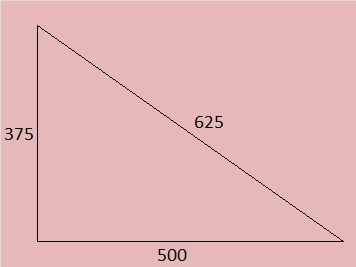625 is the hypotenuse of FOUR Pythagorean triples. Where did all those Pythagorean triples come from?

They come from 4 of the 5 factors of 625, all of which are powers of 5, a prime number of the form (4n + 1).

5 is the hypotenuse of the primitive Pythagorean triple 3-4-5 that was calculated from (2^2) – (1^2); 2(2)(1); (2^2) + (1^2).

25 is the hypotenuse of 5 times that triple plus it has a primitive of its own:

• 15-20-25 which is 5 times 3-4-5
• 7-24-25 calculated from (4^2) – (3^2); 2(4)(3); (4^2) + (3^2)

125 is the hypotenuse of 5 times 25’s two triples plus it has a primitive of its own:

• 75-100-125 which is 5 times 15-20-25 or 25 times 3-4-5
• 35-120-125 which is 5 times 7-24-25
• 44-117-125 calculated from 2(11)(2); (11^2) – (2^2); (11^2) + (2^2)

625 is the hypotenuse of 5 times 125’s three triples plus it has a primitive of its own:

• 375-500-625 which is 5 times 75-100-125, or 25 times 15-20-25, or 125 times 3-4-5
• 175-600-625 which is 5 times 35-120-125, or 25 times 7-24-25
• 220-585-625 which is 5 times 44-117-125
• 336-527-625 calculated from 2(24)(7); (24^2) – (7^2); (24^2) + (7^2)

—————————————————————————————————625 is also the short leg of FOUR Pythagorean triples. Where did all those Pythagorean triples come from?

They come from 4 of the 5 factors of 625, all of which are odd. (Every odd number greater than one is the short leg of at least one Pythagorean triple.)

5 is the short leg of the primitive Pythagorean triple 5-12-13. Notice that 12 + 13 = 25 which is 5^2.

25 is the short leg of 5 times that triple plus it has a primitive of its own:

• 25-60-65 which is 5 times 5-12-13
• 25-312-313; Notice that 312 + 313 = 625 = (25^2)

125 is the short leg of 5 times 25’s two triples plus it has a primitive of its own:

• 125-300-325 which is 5 times 25 -60-65 or 25 times 5-12-13
• 125-1560-1565 which is 5 times 25-312-313
• 125-7812-7813; Notice that 7812 + 7813 = (125^2)

625 is the short leg of 5 times 125’s three triples plus it has a primitive of its own:

• 625-1500-1625 which is 5 times 125-300-325, or 25 times 25-60-65, or 125 times 5-12-13
• 625-7800-7825 which is 5 times 125-1560-1565 or 25 times 25-312-313
• 625-39060-39065 which is 5 times 125-7812-7813
• 625-195312-195313; Notice that 195312 + 195313 = (625^2)

Thus 625 appears in 8 Pythagorean triples, and now you know where they all came from.

—————————————————————————————————

Here are some other fun facts about the number 625:

Look at this pattern:

• (5^2) = 25
• (25^2) = 625
• (625^2) = 390,625
• but (390,625^2) = 152,587,890,625 so one digit discontinued the pattern!

625 is the sum of the seven prime numbers from 73 to 103.

What would happen if we ran the following prime number tests on 625?

(24^2) + (7^2) = 625, and 24 and 7 have no common prime factors. That means that 625’s only possible prime factors less than √625 are 5, 13, and 17. Obviously 625 is divisible by 5 so it isn’t a prime number.

Also note that (20^2) + (15^2) = 625, but 20 and 15 have a common prime factor, 5. The fact that they have a common prime factor means that 625 cannot be a prime number.

—————————————————————————————————

• 625 is a composite number.
• Prime factorization: 625 = 5 x 5 x 5 x 5, which can be written 625 = 5^4
• The exponent in the prime factorization is 4. Adding one, we get (4 + 1) = 5. Therefore 625 has exactly 5 factors.
• Factors of 625: 1, 5, 25, 125, 625
• Factor pairs: 625 = 1 x 625, 5 x 125 or 25 x 25
• 625 is a perfect square and a perfect fourth power. √625 = (√25)(√25) = 25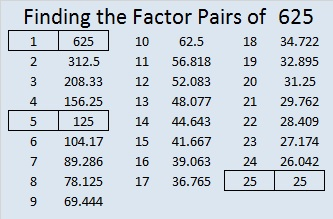# 623 and Level 2

623 is the hypotenuse of Pythagorean triple 273-560-623. What is the greatest common factor of those three numbers?

623 is not divisible by 2, 3, or 5. Is 623 divisible by 7? You can apply either of the following divisibility rules after you separate 623’s digits into 62 and 3:

1st rule: 62 – 2(3) = 62 – 6 = 56. Since 56 is divisible by 7, 623 is divisible by 7.

2nd rule: 62 + 5(3) = 62 + 15 = 77. Since 77 is divisible by 7, 623 is divisible by 7.

86 + 87 + 88 + 89 + 90 + 91 + 92 = 623  (7 consecutive numbers because 623 is divisible by 7)Print the puzzles or type the solution on this excel file: 12 Factors 2015-09-21

—————————————————————————————————

• 623 is a composite number.
• Prime factorization: 623 = 7 x 89
• The exponents in the prime factorization are 1 and 1. Adding one to each and multiplying we get (1 + 1)(1 + 1) = 2 x 2 = 4. Therefore 623 has exactly 4 factors.
• Factors of 623: 1, 7, 89, 623
• Factor pairs: 623 = 1 x 623 or 7 x 89
• 623 has no square factors that allow its square root to be simplified. √623 ≈ 24.9599679.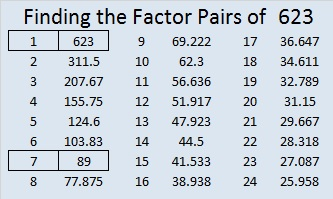—————————————————————————————————# 615 and Level 1

615 is the hypotenuse of four Pythagorean triples. Can you find the greatest common factor for each triple?

• 135-600-615
• 252-561-615
• 369-492-615
• 399-468-615Print the puzzles or type the solution on this excel file: 10 Factors 2015-09-14

—————————————————————————————————

• 615 is a composite number.
• Prime factorization: 615 = 3 x 5 x 41
• The exponents in the prime factorization are 1, 1, and 1. Adding one to each and multiplying we get (1 + 1)(1 + 1)(1 + 1) = 2 x 2 x 2 = 8. Therefore 615 has exactly 8 factors.
• Factors of 615: 1, 3, 5, 15, 41, 123, 205, 615
• Factor pairs: 615 = 1 x 615, 3 x 205, 5 x 123, or 15 x 41
• 615 has no square factors that allow its square root to be simplified. √615 ≈ 24.79919.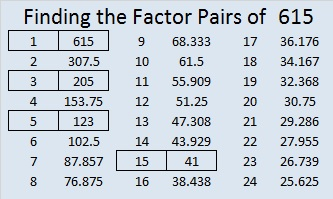—————————————————————————————————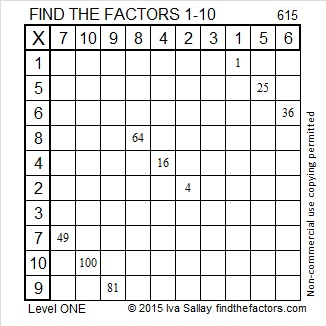# 611 and Level 4

611 is the sum of 13 consecutive numbers:

41 + 42 + 43 + 44 + 45 + 46 + 47 + 48 + 49 + 50 + 51 + 52 + 53 = 611

611 is the sum of 13 consecutive odd numbers:

35 + 37 + 39 + 41 + 43 + 45 + 47 + 49 + 51 + 53 + 55 + 57 + 59 = 611

611 is the sum of the fifteen prime numbers from 13 to 71, a set of numbers that includes all the prime factors of 611.

611 is also the hypotenuse of the Pythagorean triple 235-564-611. What is the greatest common factor of those three numbers?

If you average 13 and 47 (two odd numbers in a factor pair of 611), you get 30 which is 17 numbers away from either factor. That means that (30^2) – (17^2) = 611.

—————————————————————————————————

Today’s puzzle starts off easy enough, but it might get a bit tricky to complete.Print the puzzles or type the solution on this excel file: 12 Factors 2015-09-07

—————————————————————————————————

• 611 is a composite number.
• Prime factorization: 611 = 13 x 47
• The exponents in the prime factorization are 1 and 1. Adding one to each and multiplying we get (1 + 1)(1 + 1) = 2 x 2 = 4. Therefore 611 has exactly 4 factors.
• Factors of 611: 1, 13, 47, 611
• Factor pairs: 611 = 1 x 611 or 13 x 47
• 611 has no square factors that allow its square root to be simplified. √611 ≈ 24.718414.—————————————————————————————————# 598 and Level 6

598 is the sum of all the prime number from 17 to 71.

598 is also the hypotenuse of the Pythagorean triple 230-552-598. What is the greatest common factor of those three numbers?

Here’s another easy as 1-2-3 number fact from OEIS.org: (5^1) + (9^2) +(8^3) = 598.

Since 23 + 3 = 26 and 23 x 26 = 598, we know we are only 2 away from 24 x 25 = 600.Print the puzzles or type the solution on this excel file: 12 Factors 2015-08-24

—————————————————————————————————

• 598 is a composite number.
• Prime factorization: 598 = 2 x 13 x 23
• The exponents in the prime factorization are 1, 1, and 1. Adding one to each and multiplying we get (1 + 1)(1 + 1)(1 + 1) = 2 x 2 x 2 = 8. Therefore 598 has exactly 8 factors.
• Factors of 598: 1, 2, 13, 23, 26, 46, 299, 598
• Factor pairs: 598 = 1 x 598, 2 x 299, 13 x 46, or 23 x 26
• 598 has no square factors that allow its square root to be simplified. √598 ≈ 24.4540385.—————————————————————————————————# 596 Select the Correct Top Row

596 is the sum of the four primes numbers from 139 to 157. The two prime numbers in the middle of that sum are twin primes. Do you know what they are?

596 is also the hypotenuse of the Pythagorean triple 204-560-596. What is the greatest common factor of those three numbers?Print the puzzles or type the solution on this excel file: 12 Factors 2015-08-24

—————————————————————————————————

• 596 is a composite number.
• Prime factorization: 596 = 2 x 2 x 149, which can be written 596 = (2^2) x 149
• The exponents in the prime factorization are 1 and 2. Adding one to each and multiplying we get (1 + 1)(2 + 1) = 2 x 3  = 6. Therefore 596 has exactly 6 factors.
• Factors of 596: 1, 2, 4, 149, 298, 596
• Factor pairs: 596 = 1 x 596, 2 x 298, or 4 x 149
• Taking the factor pair with the largest square number factor, we get √596 = (√4)(√149) = 2√149 ≈ 24.41311123—————————————————————————————————# 593 and Level 1

593 is the sum of the seven prime numbers from 71 to 101 as well as the nine prime numbers from 47 to 83.

When the number formed from the last two digits of prime number 593 is divided by 4, the remainder is 1 so 593 can be expressed at the sum of two squares AND is the hypotenuse of a primitive Pythagorean triple. Specifically:

23² + 8² = 593

593 is the hypotenuse of the primitive Pythagorean triple prime 368-465-593Print the puzzles or type the solution on this excel file: 12 Factors 2015-08-24

—————————————————————————————————

• 593 is a prime number.
• Prime factorization: 593 is prime.
• The exponent of prime number 593 is 1. Adding 1 to that exponent we get (1 + 1) = 2. Therefore 593 has exactly 2 factors.
• Factors of 593: 1, 593
• Factor pairs: 593 = 1 x 593
• 593 has no square factors that allow its square root to be simplified. √593 ≈ 24.35159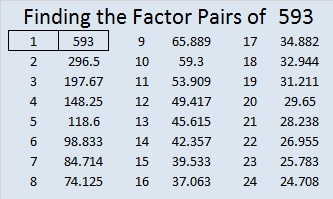How do we know that 593 is a prime number? If 593 were not a prime number, then it would be divisible by at least one prime number less than or equal to √593 ≈ 24.4. Since 593 cannot be divided evenly by 2, 3, 5, 7, 11, 13, 17, 19, or 23, we know that 593 is a prime number.

—————————————————————————————————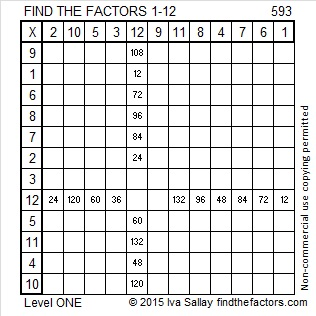# 592 and The Broken Internet Connection

There is a lot of road construction near my house. I don’t know if it is related, but the outside cable that provides us internet connection died sometime on the morning of Sunday, 23 August 2015.

That broken cable line meant that we had no home phone service, no internet, no banking information, no twitter, no facebook = no looking at the latest pictures of my grandchildren, no reading Blogspot, no watching Netflix or Youtube, and no reading or writing on WordPress.

I don’t have a smart phone, but my husband uses the Wifi in our house for his. His smart phone wasn’t smart enough to switch to our phone’s data plan. Instead it relentlessly tried to connect to the Wifi every time either of us tried to use it.

My dead internet connection meant that if you were looking for information about the number 592 (and who isn’t), you had to wait a few days:

___________________________________

592 is a palindrome in three bases:

• 727 BASE 9 because 7(81) + 2(9) + 7(1) = 592
• 414 BASE 12 because 4(144) + 1(12) + 4(1) = 592
• GG BASE 36 (G is 16 in base 10) because 16(36) + 16(1) = 592

592 is the hypotenuse of the Pythagorean triple 192-560-592. Which factor of 592 is the greatest common factor of those three numbers?

• 592 is a composite number.
• Prime factorization: 592 = 2 x 2 x 2 x 2 x 37, which can be written 592 = (2^4) x 37
• The exponents in the prime factorization are 4 and 1. Adding one to each and multiplying we get (4 + 1)(1 + 1) = 5 x 2 = 10. Therefore 592 has exactly 10 factors.
• Factors of 592: 1, 2, 4, 8, 16, 37, 74, 148, 296, 592
• Factor pairs: 592 = 1 x 592, 2 x 296, 4 x 148, 8 x 74, or 16 x 37
• Taking the factor pair with the largest square number factor, we get √592 = (√16)(√37) = 4√37 ≈ 24.33105# 591 and a Sometimes Impossible Level 6

5 + 9 + 1 = 15, a multiple of 3 so 591 is divisible by 3.

However, since 9 is a multiple of 3, it isn’t necessary to include it in the equation, so I simple say 5 + 1 = 6, a multiple of 3 so 591 is divisible by 3.

591 is the hypotenuse of the Pythagorean triple 84-585-591. Which of 591’s factors is the greatest common factor of those three numbers?

—————————————————————————————————

I love that Joseph Nebus wrote that the FIND THE FACTORS puzzles are “a fun recreational mathematics puzzle even if the level 6’s and sometimes level 5’s will sometimes feel impossible.”

Judge for yourself. Is this Level 6 puzzle impossible or not!Print the puzzles or type the solution on this excel file: 10 Factors 2015-08-17

—————————————————————————————————

• 591 is a composite number.
• Prime factorization: 591 = 3 x 197
• The exponents in the prime factorization are 1 and 1. Adding one to each and multiplying we get (1 + 1)(1 + 1) = 2 x 2 = 4. Therefore 591 has exactly 4 factors.
• Factors of 591: 1, 3, 197, 591
• Factor pairs: 591 = 1 x 591 or 3 x 197
• 591 has no square factors that allow its square root to be simplified. √591 ≈ 24.31049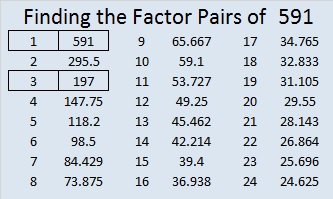—————————————————————————————————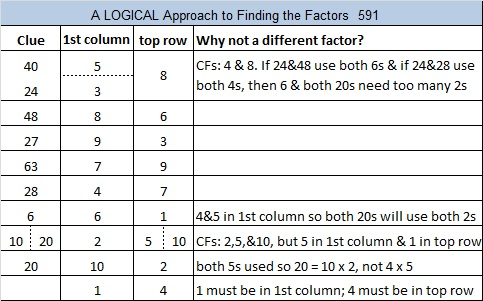# 586 and Level 1

586 is the hypotenuse of the Pythagorean triple 136-570-586. Which factor of 586 is the greatest common factor of those three numbers?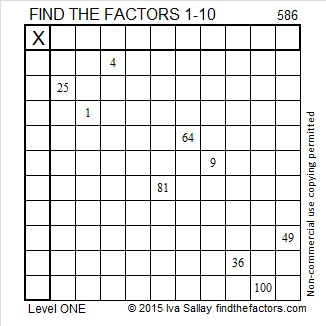Print the puzzles or type the solution on this excel file: 10 Factors 2015-08-17

—————————————————————————————————

• 586 is a composite number.
• Prime factorization: 586 = 2 x 293
• The exponents in the prime factorization are 1 and 1. Adding one to each and multiplying we get (1 + 1)(1 + 1) = 2 x 2 = 4. Therefore 586 has exactly 4 factors.
• Factors of 586: 1, 2, 293, 586
• Factor pairs: 586 = 1 x 586 or 2 x 293
• 586 has no square factors that allow its square root to be simplified. √586 ≈ 24.2074—————————————————————————————————